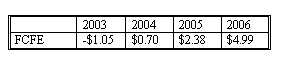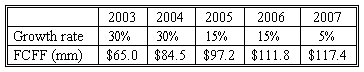### Seeing is believing!

Before you order, simply sign up for a free user account and in seconds you'll be experiencing the best in CFA exam preparation.

##### Subject 5. Free cash flow model variations
Summary

Point: Match growth pattern to model.

• Single-stage, stable growth.
• Two-stage model.

• Fixed growth in stages 1 and 2.
• Declining growth in stage 1.

• Three-stage model.

• Fixed growth in all three stages.
• Declining growth in stage 2.

Single-Stage Free Cash Flow Models

Single-stage FCFF model

This model is employed when free cash flow to the firm is expected to grow at a stable rate indefinitely extending in the future.

Firm value = FCFF0 (1 + g) / (WACC - g) = FCFF1/(WACC - g)

Equity value = Firm value - Market value of debt

Single-stage FCFE model

This model is employed when free cash flow to equity is expected to grow at a stable rate indefinitely extending in the future. This rate may not be the same as the rate in the previous model.

Equity value = FCFE0 (1 + g)/(r - g) = FCFF1/(r - g)

Note:

• Income stock (low, constant growth).
• International setting or volatile inflation rates: use real rates.

Example

• FCFF = \$600,000.
• Target debt ratio = 30%.
• MV of the firm's debt = \$3,530,000.
• 500,000 shares of common stock.
• Tax rate = 40%.
• Required return on equity = 14%.
• Cost of debt = 9%.
• Expected growth rate in FCFF = 6%.

Calculate the value of the firm.

WACC = (0.70 x 0.14) + [0.30 x 0.09 x (1 - 0.40)] = 0.114 = 11.4%.
Firm value = (\$600,000 x 1.060) / (0.114 - 0.060) = \$11,777,778.
Equity value = \$11,777,778 - \$3,530,000 = \$8,247,778.
Equity per share = \$8,247,778 / 500,000 = \$16.50.

Two-Stage Free Cash Flow Models

These models are employed when a company's growth is expected to decelerate in the future and stabilize at some rate g. Another case when the two-stage models are appropriate is when the growth is stable but is expected to drop discontinuously to another stable rate at some point in the future. The growth trends described by the two-stage model can occur if a company is losing its competitive advantage or the entire industry is entering the mature stage. It is worth noting, that the growth rates do not necessarily describe dynamics of free cash flows. Analysts may use growth rates of sales, earnings, or some other fundamentals and then forecast growth rates of free cash flows.

Two-stage FCFF model

At the beginning of the stable-growth stage (for example, at the beginning of period n), the terminal firm value can be calculated using the single-stage model:

Terminal Firm Value = FCFFn+1 / (WACC - g)

To find the total present value of a firm, we discount FCFF before the terminal period and the terminal firm value, using WACC as the discount rate:

Firm value = Σ (FCFFt / (1 + WACC)t) + FCFFn+1 / [(WACC - g) (1+WACC)n]
Σ: t = 1 to n.

Equity value = Firm value - Market value of debt

Two-stage FCFE model

Terminal value of equity is:

Terminal Firm Value = FCFEn+1/(r - g)

Similarly to the DDM model, an alternative way to estimate the terminal value of equity is to find a product of a price multiple and expected sales, earnings or cash flow. To find the equity value, we discount terminal equity value and expected free cash flows to equity before the terminal period, using the cost of equity as the discount rate:

Equity value = Σ (FCFEt / (1 + r)t) + FCFEn+1/[(r - g) (1 + r)n]
Σ: t = 1 to n.

Example

• 2002 EPS = \$3.00.
• Target debt ratio = 20%
• Equity required return = 16%
• Growth rate beyond 2006 = 4%TV2005 = \$4.99/(0.16 - 0.04) = \$41.58.
V2002 = -\$1.05/1.161 + 0.70/1.162 + (\$2.38 + \$41.58)/1.163 = \$27.78.

Both the two-stage DDM and FCFE models allow for two distinct phases of growth, an initial finite period where the growth is abnormal, followed by a stable growth period that is expected to last forever. These two-stage models share the same limitations with respect to the growth assumptions:

• There is the difficulty of defining the duration of the extraordinary growth period. For example, a longer period of high growth will lead to a higher valuation, and analysts may be tempted to assume an unrealistically long period of extraordinary growth.
• An assumption of a sudden shift from high growth to lower, stable growth is unrealistic. The transformation more likely will occur gradually over a period of time.
• Because value is quite sensitive to the steady growth assumption, overestimating or underestimating this rate can lead to large errors in value.
• The two models share other limitations as well, notably difficulties in accurately estimating required rates of return.

Three-Stage Free Cash Flow Models

These models are used in two general cases:

• When growth rate is constant in three stages;
• When free cash flows grow at a stable rate in the first stage, then the growth falls gradually until it stabilizes at some constant rate.

Three-stage models are particularly useful for valuing companies that are currently in the growth phase of their industry life cycle. The three stages of the model then coincide with growth phase, transitional phase and maturity phase.

Calculation is similar to the two-stage model: determine the terminal value (at the beginning of mature growth stage) and discount it together with all preceding free cash flows to the present.

Example

• FCFF 2002 = \$50 million.
• Market value of debt = \$300 million.
• Shares outstanding = 40 million.
• Growth rates:

• 2003-2004: 30%.
• 2005-2006: 15%
• After 2006: 5%.

• WACC = 12%.
• Cost of equity = 15%.

Compute equity value.TV2006 = \$117.4/(0.12 - 0.05) = \$1,677 million.
V2002 = \$65.0/1.121 + \$84.5/1.122 + \$97.2/1.123 + (\$111.8 + \$1,677)/1.124 = \$1,331.4 million.
Equity = \$1,331.4 - \$300 = \$1,031.4 million.
Equity value per share = \$1,031.4 / 40 = \$25.78.

Sensitivity Analysis

Just like with any other valuation technique, inputs determine the quality of valuation performed by FCF models. Few analysts are absolutely confident in their assumptions. Frequently they use sensitivity analysis to determine the value of the firm or equity by changing the values of inputs. A sensitivity analysis shows how sensitive the final valuation is to changes in each of a valuation model's input variables.

Two major sources of error in valuation analysis:

• Estimating the future growth in FCFF and FCFE.
• The base-years for the FCFF or FCFE growth forecasts are critical.